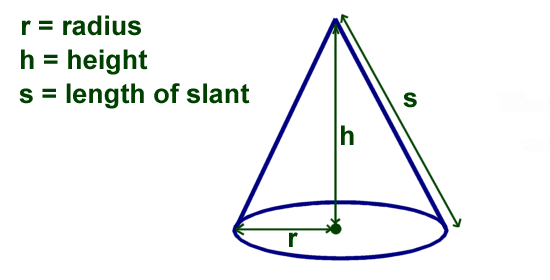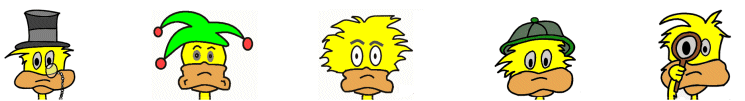# Kids Math

## Finding the Volume and Surface Area of a Cone

What is a cone?

A cone is a type of geometric shape. There are different kinds of cones. They all have a flat surface on one side that tapers to a point on the other side.

We will be discussing a right circular cone on this page. This is a cone with a circle for a flat surface that tapers to a point that is 90 degrees from the center of the circle.Terms of a Cone

In order to calculate the surface area and volume of a cone we first need to understand a few terms:

Radius - The radius is the distance from the center to the edge of the circle at the end.

Height - The height is the distance from the center of the circle to the tip of the cone.

Slant - The slant is the length from the edge of the circle to the tip of the cone.

Pi - Pi is a special number used with circles. We will use an abbreviated version where Pi = 3.14. We also use the symbol π to refer to the number pi in formulas.

Surface Area of a Cone

The surface area of a cone is the surface area of the outside of the cone plus the surface area of the circle at the end. There is a special formula used to figure this out.

Surface area = πrs + πr2

s = slant
π = 3.14

This is the same as saying (3.14 x radius x slant) + (3.14 x radius x radius)

Example:

What is the surface area of a cone with radius 4 cm and slant 8 cm?

Surface area = πrs + πr2
= (3.14x4x8) + (3.14x4x4)
= 100.48 + 50.24
= 150.72 cm2

Volume of a Cone

There is special formula for finding the volume of a cone. The volume is how much space takes up the inside of a cone. The answer to a volume question is always in cubic units.

Volume = 1/3πr2h

This is the same as 3.14 x radius x radius x height ÷ 3

Example:

Find the volume of a cone with radius 4 cm and height 7 cm?

Volume = 1/3πr2h
= 3.14 x 4 x 4 x 7 ÷ 3
= 117.23 cm 3

Things to Remember
• Surface area of a cone = πrs + πr2
• Volume of a cone = 1/3πr2h
• The slant of a right circle cone can be figured out using the Pythagorean Theorem if you have the height and the radius.
• Answers for volume problems should always be in cubic units.
• Answers for surface area problems should always be in square units.

More Geometry Subjects

Circle
Polygons
Triangles
Pythagorean Theorem
Perimeter
Slope
Surface Area
Volume of a Box or Cube
Volume and Surface Area of a Sphere
Volume and Surface Area of a Cylinder
Volume and Surface Area of a Cone
Angles glossary
Figures and Shapes glossary

Back to Kids Math

Back to Kids Study